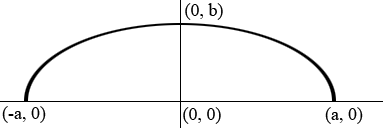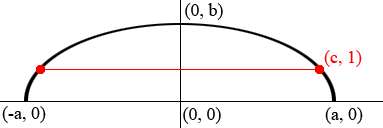SEARCH HOMEMath Central Quandaries & QueriesQuestion from Anggelica: an arch in the form of a semi-ellipse is 8 feet wide at the base and has a height of 4ft. how wide is the arch 1foot above the base?Hi,

I put the ellipse with its center at $(0, 0)$ with its major axis the X-axis and its minor axis the Y-axis. The ellipse then has equation

$\frac{x^2}{a^2} + \frac{y^2}{b^2} = 1.$Since the height of the arch is 4 feet we know that $b = 4$ feet. The width of the base is 8 feet so what is the value of $a?$ What is the equation of the ellipse?

Now I identified a point on the semi-ellipse which is 1 foot above the base.Use the equation of the ellipse to find the value of $c.$

PennyMath Central is supported by the University of Regina and The Pacific Institute for the Mathematical Sciences.